﻿ 一种用于机械臂拟人化控制的学习框架
 机器人2023, Vol. 45Issue (5): 513-5220

ZHAO Liang, YANG Tie, YU Peng, YANG Yang. A Learning Framework for Controlling Robotic Manipulators with Human-like Actions[J]. ROBOT, 2023, 45(5): 513-522.1. 中国科学院沈阳自动化研究所机器人学国家重点实验室, 辽宁 沈阳 110016;
2. 中国科学院机器人与智能制造创新研究院, 辽宁 沈阳 110169;
3. 中国科学院大学, 北京 100049

A Learning Framework for Controlling Robotic Manipulators with Human-like Actions
ZHAO Liang1,2,3, YANG Tie1,2 , YU Peng1,2 , YANG Yang1,2
1. State Key Laboratory of Robotics, Shenyang Institute of Automation, Chinese Academy of Sciences, Shenyang 110016, China;
2. Institutes for Robotics and Intelligent Manufacturing, Chinese Academy of Sciences, Shenyang 110169, China;
3. University of Chinese Academy of Sciences, Beijing 100049, China
Abstract: Endowing robotic manipulators with human-like actions can make their behaviours more explainable and predictable, which improves the quality and safety of human-robot collaboration (HRC). A human-in-the-loop learning framework is proposed to learn the human-like posture features from human teleoperation demonstration. The learnt feature model can be used to generate human-like actions on the redundant manipulators. Moreover, an online relabeling approach is adopted in the training session to address the covariate shift problem, which reduces the total demonstration time to 10 min or less. The effectiveness of the human-like control method trained by the proposed framework is validated through comparing the posture between the human and the robot, and tracking the dynamic trajectories. A user study shows that, manipulators controlled with the proposed human-like posture constraint method are more friendly to the users in subjective feelings, and more acceptable as co-operative tools for non-professional users.
Keywords: human-like control    behavioral cloning    human-robot interaction    teleoperation    exoskeleton

1 引言（Introduction）

AVATAR系统[8-10]可以将实时捕捉到的人体运动映射到一个虚拟化身或机器人中，从而在人工载体上实现拟人化动作，这是一种非常直觉化的方法。尤其在冗余结构的人形机械臂中，由于人－机运动学结构的近似性，得到拟人化的动作相对容易[11-12]。在一些已有的研究工作中，曾使用外骨骼[13-14]或基于IMU（惯性测量单元）和光学原理的运动捕捉设备来获取人体手臂的姿态。已有多项研究工作[10, 13-14, 16]基于遥操作接口，将实时捕捉到的人体手臂姿态融入到机械臂的控制量，实现机械臂的拟人化动作。然而，现实中大多数通用型机械臂并不具有类人化的结构；另一方面，机器人通常作为一种自动化工具参与人机协作，需要建立人类动作特征模型，使机器人能够自主产生拟人化的动作。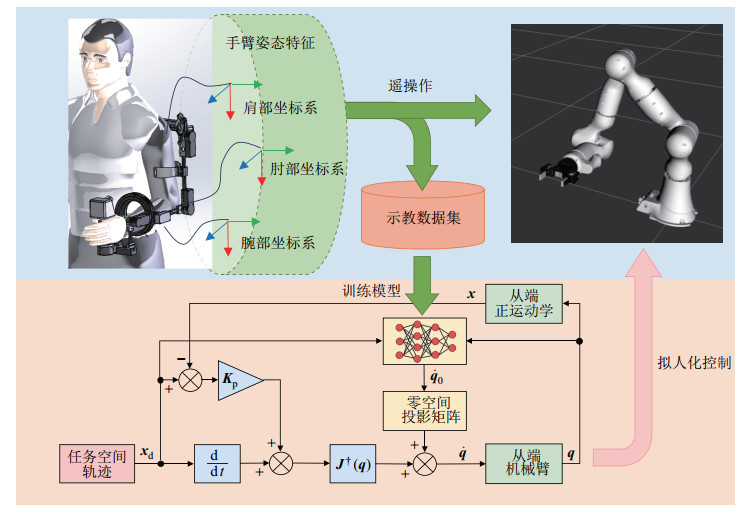图 1 机械臂拟人化控制学习框架 Fig.1 Learning framework for controlling robotic manipulators with human-like actions
2 具有姿态模仿特性的遥操作示教系统（Posture-mimicry-featured teleoperation system for demonstration） 2.1 用于动作捕捉的外骨骼主端设备

2.2 遥操作中的从端机械臂姿态约束方法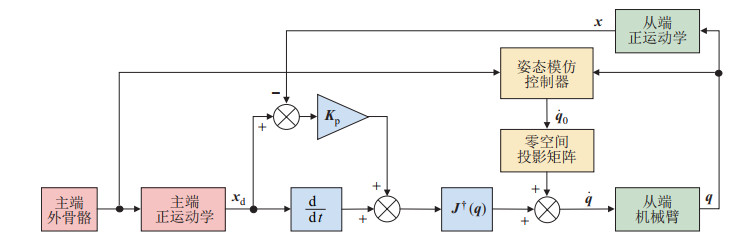图 3 具有姿态模仿特性的CLIK控制框图 Fig.3 CLIK control scheme with posture-mimicry feature

CLIK算法本质上是一种关节空间速度层面的控制策略。在冗余机械臂上，可以产生关节空间速度的CLIK控制策略可以表示为

 \begin{align} \mathit{\boldsymbol{\dot{q}}}= \mathit{\boldsymbol{J}} ^{\dagger} ( \mathit{\boldsymbol{q}} ) \left[\mathit{\boldsymbol{\dot{x}}}_{\rm d} +{\mathit{\boldsymbol{K}}}_{\rm p} ( \mathit{\boldsymbol{x}} _{\rm d} -{\mathit{\boldsymbol{x}}}) \right]+\left( \mathit{\boldsymbol{I}} - \mathit{\boldsymbol{J}} ^{\dagger} ( \mathit{\boldsymbol{q}} ) \mathit{\boldsymbol{J}} ( \mathit{\boldsymbol{q}} )\right)\mathit{\boldsymbol{\dot{q}}}_{0} \end{align} (2)

 \begin{align} \mathit{\boldsymbol{\dot{q}}}_{0} =-\eta \nabla \mathit{\boldsymbol{H}}(\mathit{\boldsymbol{q}}), \quad \nabla \mathit{\boldsymbol{H}}(\mathit{\boldsymbol{q}}) =\left(\frac{\partial \mathit{\boldsymbol{H}}(\mathit{\boldsymbol{q}})}{\partial \mathit{\boldsymbol{q}}}\right)^{\rm T} \end{align} (3)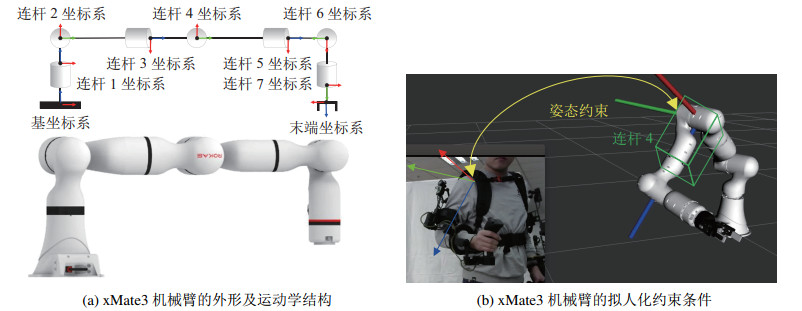图 4 从端运动学结构及其拟人化姿态约束条件 Fig.4 Kinematic structure of the slave device and its human-like posture constraints

 \begin{align} H(\mathit{\boldsymbol{q}})=\delta_{x}^{2} +\delta_{y}^{2} +\delta_{z}^{2} \end{align} (4)

 \begin{align} \mathit{\boldsymbol{S}} (\mathit{\boldsymbol{\delta}} _{\Theta} ) =\begin{bmatrix} 0 & -\delta_{z} & \delta_{y} \\ \delta_{z} & 0 & -\delta_{x} \\ -\delta_{y} & \delta_{x} & 0 \end{bmatrix} \end{align} (5)

 \begin{align} \mathit{\boldsymbol{S}}(\mathit{\boldsymbol{\delta}}_{\Theta} ) ={}_{\rm m}^{0} \mathit{\boldsymbol{R}}_{3} {}_{\rm s}^{0} \mathit{\boldsymbol{R}}_{4}^{\rm T}-\mathit{\boldsymbol{I}}_{3\times 3} \end{align} (6)

 $\underset{\theta}{\arg \min } E_{(s, a) \sim \mathcal{D}}\left(\pi_\theta-a\right)^2$ (7)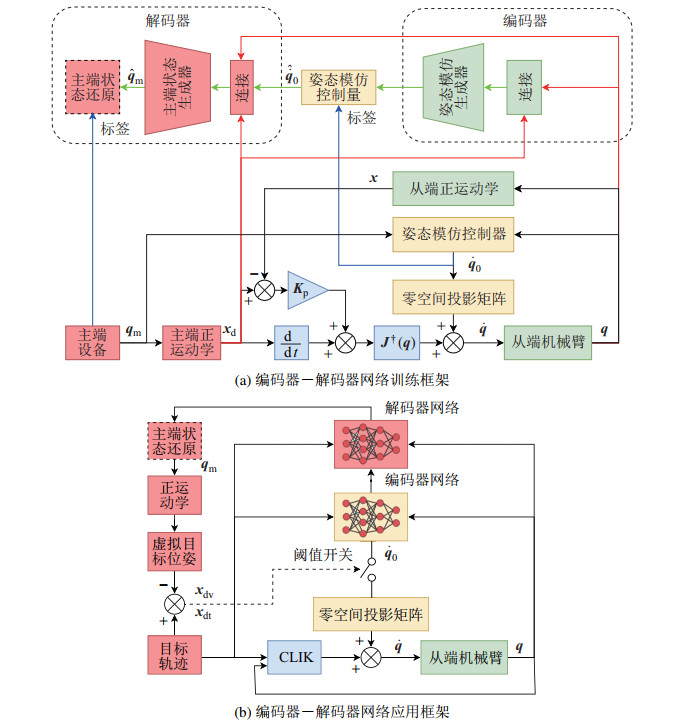图 5 编码器－解码器网络的训练和应用框架 Fig.5 Training and application frameworks of the encoder-decoder network表 1 网络结构 Tab. 1 Network structure
3.2 “人在回路”的在线再标注方法

 算法1  在线再标注 前置条件:   预训练示教数据集${\mathcal D}$                     使用数据集${\mathcal D}$预训练的策略$\pi_{\theta}$1: $\mathcal{D}_{\mathrm{E}} \leftarrow \varnothing$2: For 再标注轮次$i = 1, 2, \cdots, n _{\text{round}}$ do3:     For 时间点$t = 1, 2, \cdots, n _{\text{steps}}$ do                       // 使用策略产生拟人化姿态约束控制量4:            $\mathord{\buildrel{\lower3pt\hbox{$\scriptscriptstyle\frown$}} \over {\boldsymbol{a}}} _t \leftarrow \pi_\theta\left(\boldsymbol{s}_t\right)$                       // 通过遥操作获取姿态约束控制量5:             ${\mathit{\boldsymbol{a}}}_{t} \xleftarrow{\text{teleoperation}} \pi_{h} ({\mathit{\boldsymbol{s}}}_{t})$6:             If$\left\|\boldsymbol{a}_t-\mathord{\buildrel{\lower3pt\hbox{$\scriptscriptstyle\frown$}} \over {\boldsymbol{a}}} _t\right\|^2 \geqslant \varepsilon$ then7:                      ${\mathcal D}_{\rm E} \leftarrow {\mathcal D}_{\rm E} \cup ({\mathit{\boldsymbol{s}}}_{t}, {\mathit{\boldsymbol{a}}}_{t})$8:             end if9:     end for                       // 合并再标注的数据集10:    ${\mathcal D}\leftarrow {\mathcal D}_{\rm E} \cup {\mathcal D}$                       // 执行再训练11:    for each $({\mathit{\boldsymbol{s}}}_{t}, {\mathit{\boldsymbol{a}}}_{t})\sim {\mathcal D}$ do12:            $\theta_{n} \leftarrow \arg\min\limits_{\theta} \| \pi_{\theta} ({\mathit{\boldsymbol{s}}}_{t})-{\mathit{\boldsymbol{a}}}_{t} \|$13:    end for                       // 更新策略网络参数14:    $\pi_{\theta} \leftarrow \theta_{n}$15: end for

4 实验（Experiments） 4.1 实验环境

4.2 在线再标注方法效果评价测试表 2 示教和再标注时间参数 Tab. 2 Temporal parameters of demonstration and relabeling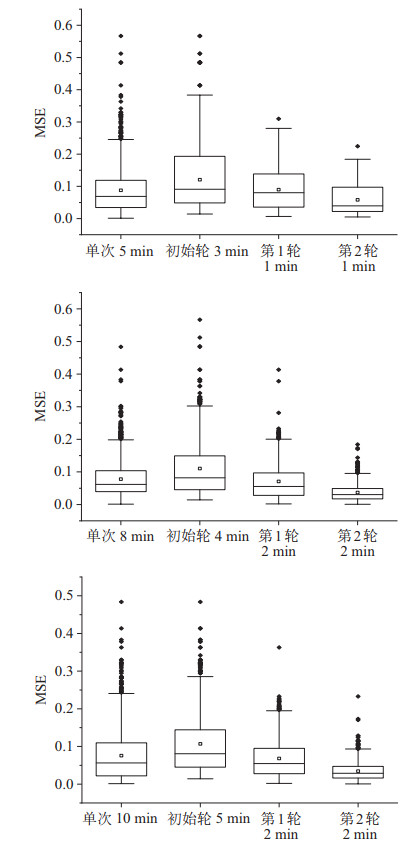图 6 使用不同示教与再标注时间参数训练的模型误差 Fig.6 Error of models trained with different temporal parameters of demonstration and relabeling

4.3 拟人化姿态约束有效性测试及用户评价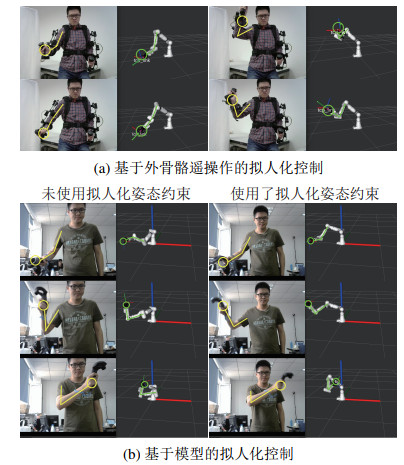图 7 拟人化姿态控制有效性测试 Fig.7 Effectiveness test of the human-like posture control表 3 用户评价统计结果 Tab. 3 Statistic results of user study
4.4 机械臂拟人化控制动态性能测试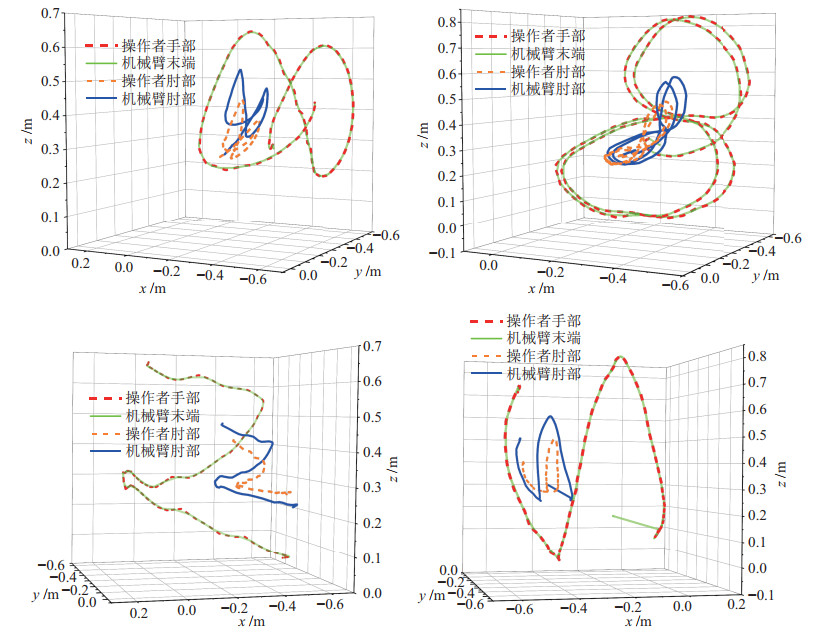图 8 动态跟踪任务中关键点轨迹 Fig.8 Trajectories of key points in dynamic tracking tasks

5 结论（Conclusion）